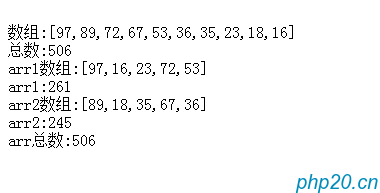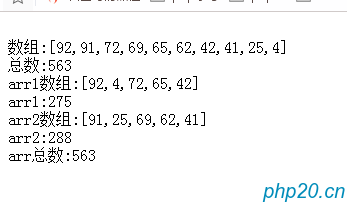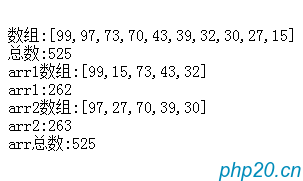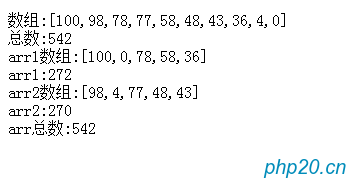# php关于数组n个随机数分成x组,使每组值相近的算法

```<?php

function group_arr(\$arr_count, \$max_num)
{
\$arr = array();
for (\$i = 0; \$i < \$arr_count; \$i++) {
\$arr[] = mt_rand(0, \$max_num);
}
rsort(\$arr);
//var_dump(\$arr);
//从大到小排序一下
\$total = array_sum(\$arr);
//var_dump(\$total);
\$arr1     = array();
\$arr2     = array();
\$arr1_sum = 0;
\$arr2_sum = 0;
\$mean     = \$total / 2;//平均数
\$arr_arr = \$arr;
for (\$i = 0; \$i < \$arr_count / 2; \$i++) {
//        var_dump(\$arr_arr);
if (\$arr1_sum > \$arr2_sum) {
\$arr1_sum += \$arr1[] = \$arr_arr[count(\$arr_arr)-1];
array_splice(\$arr_arr,-1,1);//数组删除元素重排
\$arr2_sum += \$arr2[] = \$arr_arr[count(\$arr_arr)-1];
array_splice(\$arr_arr,-1,1);//数组重排
} else {
\$arr1_sum += \$arr1[] = \$arr_arr;
array_splice(\$arr_arr,0,1);//数组重排
\$arr2_sum += \$arr2[] = \$arr_arr;
array_splice(\$arr_arr,0,1);//数组重排
}
}
echo '<br>数组:' . json_encode(\$arr);
echo '<br>总数:' . array_sum(\$arr);
echo '<br>arr1数组:' . json_encode(\$arr1);
echo '<br>arr1:' . array_sum(\$arr1);
echo '<br>arr2数组:' . json_encode(\$arr2);
echo '<br>arr2:' . array_sum(\$arr2);
echo '<br>arr总数:' .( array_sum(\$arr1)+array_sum(\$arr2));

}

group_arr(10, 100);```## phpunit-单元测试神器

2022-3-2 16:27:10

## 百度编辑器-easyswoole组件版

2022-3-2 16:51:47